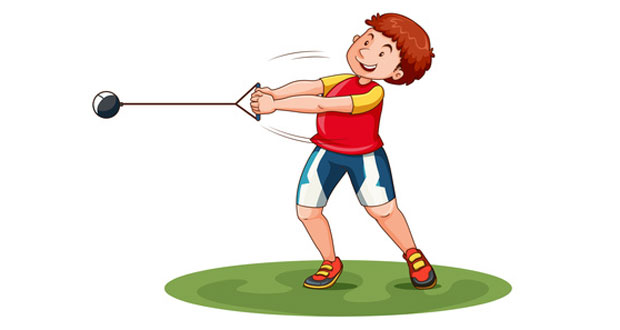# Angular Momentum In SportAngular momentum can be defined as the movement of a mass when it is rotating or spinning. You may have seen a situation when a person in a tucked position spins faster or than someone in an extended position. Here we explane angular momentum in sport.

To explain the movement of a mass when it is rotating, we must first understand angular momentum. This is the movement of a mass around an object and is calculated by multiplying angular velocity with the moment of inertia.

Angular momentum = angular velocity x moment of inertia

## Angular velocity

Angular velocity is referred to as the speed of rotation. Or in other words, how quickly something rotates.

## Moment of inertia

The moment of inertia is referred to as the resistance of a body to change state when rotating. In other words, its reluctance to rotate. If a body has a high reluctance to rotate (high moment of inertia), it would have a lower angular velocity.  If a mass has a low resistance to rotate, then it will have a higher angular velocity.

The moment of inertia can be calculated by multiplying the centre of mass x distance from the axis.

Moment of inertia = mass x distance from the axis of rotation

A body is unlikely to lose mass during a movement but it can change its distance or distribution from the axis of rotation. If the mass moves closer to the axis of rotation then the moment of inertia decreases, resulting in the angular velocity increasing. If a mass moves further away from the axis of rotation, then the moment of inertia increases and angular momentum decreases.

In image one below, the ice skater is in an extended shape, increasing the moment of inertia, in this circumstance the ice skater will rotate but will do so at a slower rate. In image two, the ice skater has tucked her arms and legs inwards, decreasing the moment of inertia, resulting in an increased speed of rotation.